# Interference and the Two-slit Experiment

• The Principle of Superposition states that when several light waves coincide, the result is equal to the sum of the individual waves. Phases are important!
• If two light waves of same wavelength and phase travel different distances to reach the same point, their interference will be
• constructive if difference in path length is integer number of wavelengths
• destructive if difference in path length is half-integer number of wavelengths
• The double-slit experiment forces light waves to interfere on a distant wall after travelling different distances
• Consider a point on the distant wall a distance y = L sin(theta) away from the center point. Light will interfere
• constructively (light spot) if
```               d sin(theta) = m lambda               m = 0, 1, 2, ...
```
• destructively (dark spot) if
```               d sin(theta) = (m + 1/2) lambda       m = 0, 1, 2, ...
```
where d is the separation of the two slits, and lambda is the wavelength of the light.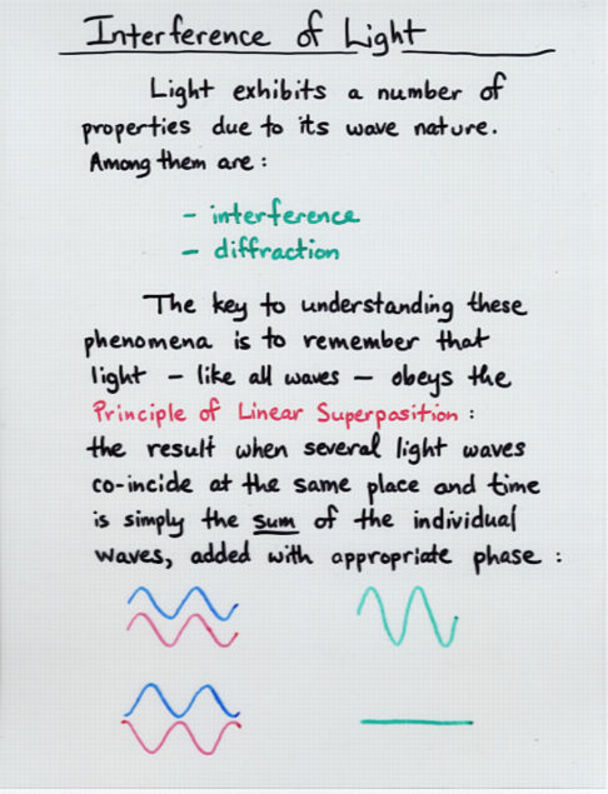Viewgraph 1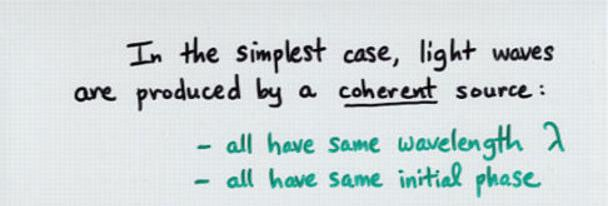Viewgraph 2a

```

Q:  What type of light source produces
coherent light waves?

```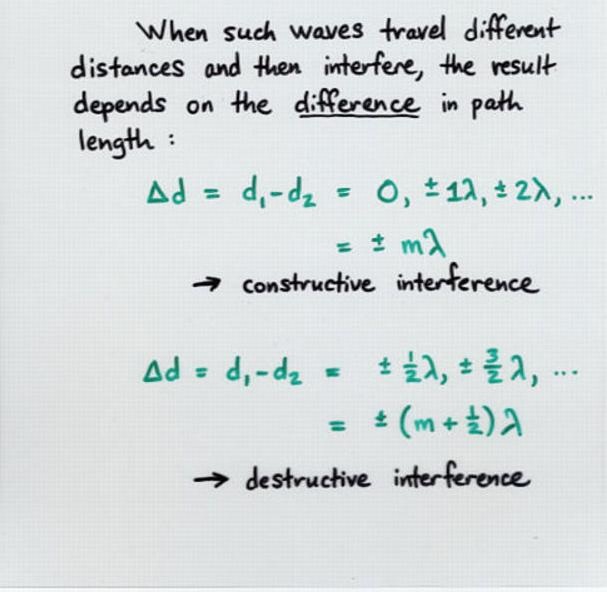Viewgraph 2b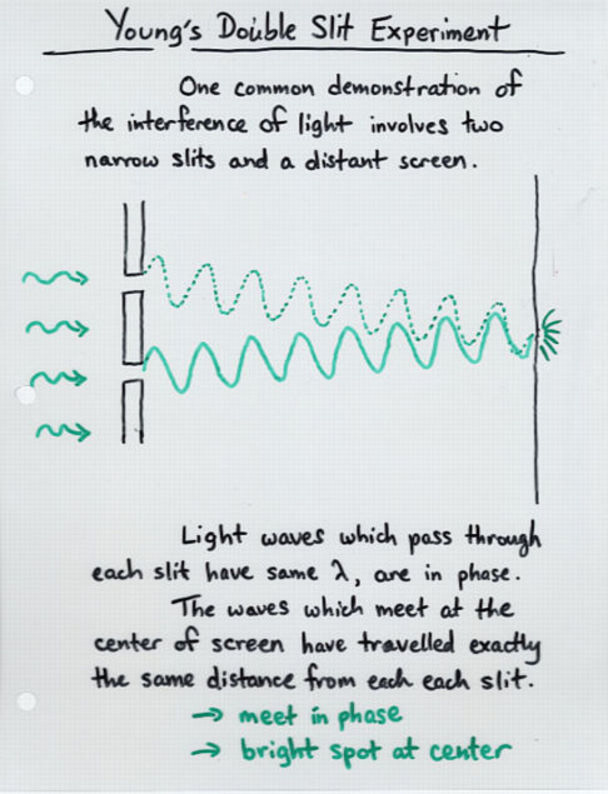Viewgraph 3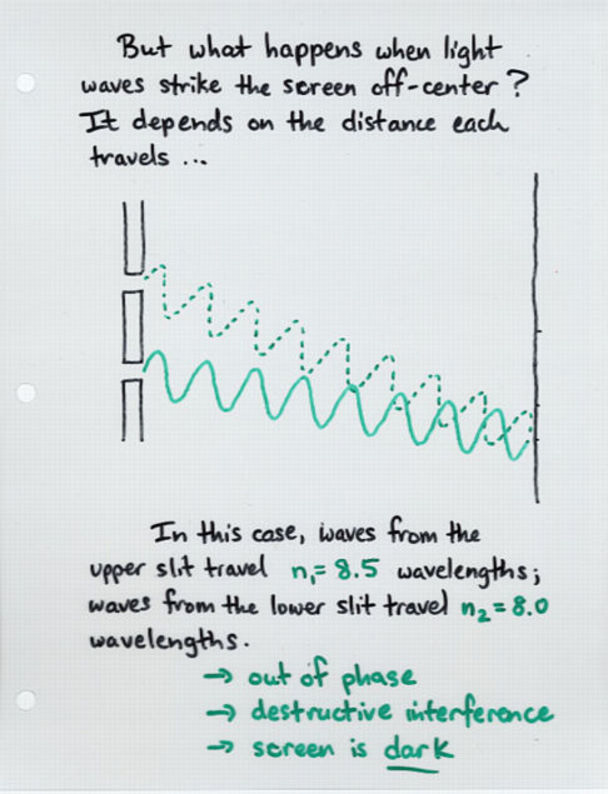Viewgraph 4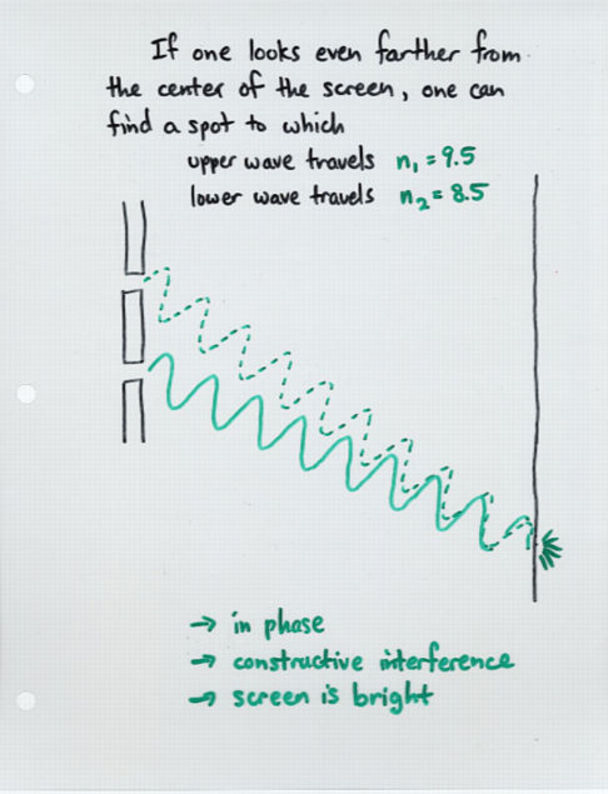Viewgraph 5Viewgraph 6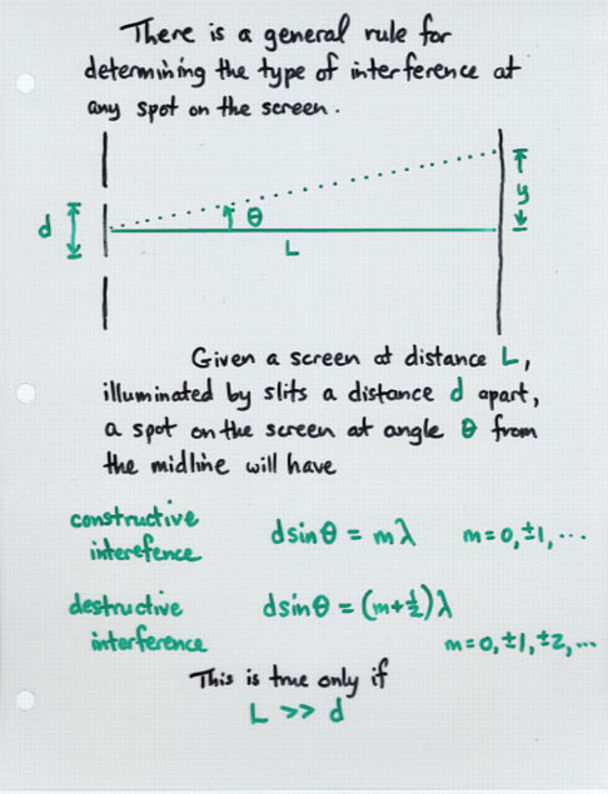Viewgraph 7

```

Joe shines a blue light of wavelength 450 nm
through a pair of slits which are 0.2 mm apart.

Q:  Find three angles at which Joe will find
a bright spot on the distant wall.

Q:  Find three angles at which Joe will find
a dark spot on the distant wall.

```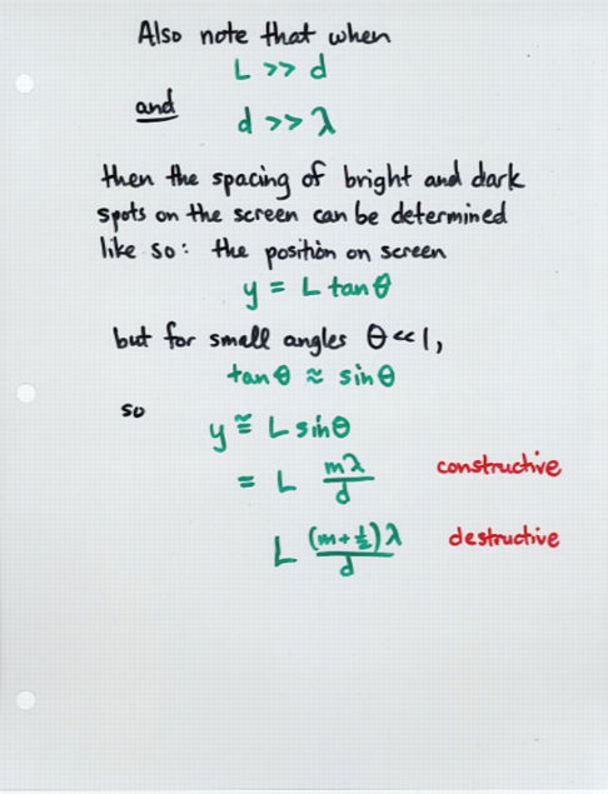Viewgraph 8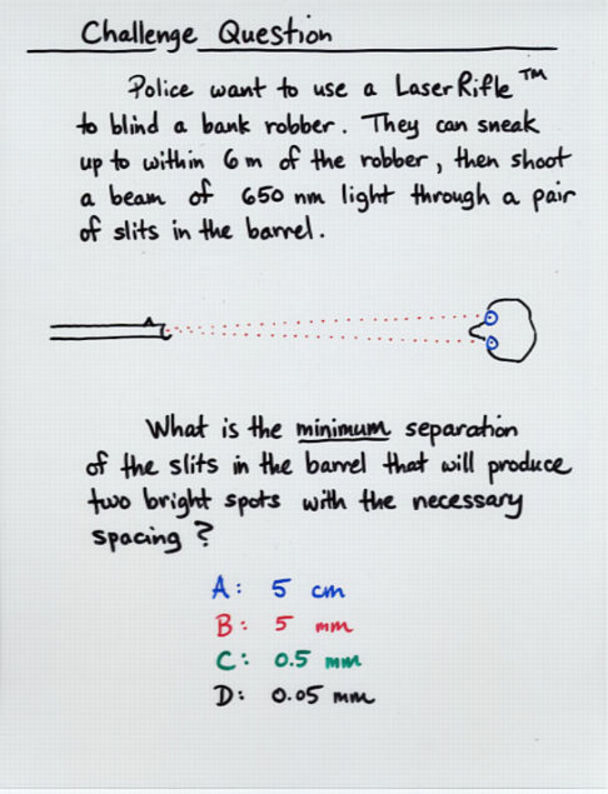Viewgraph 9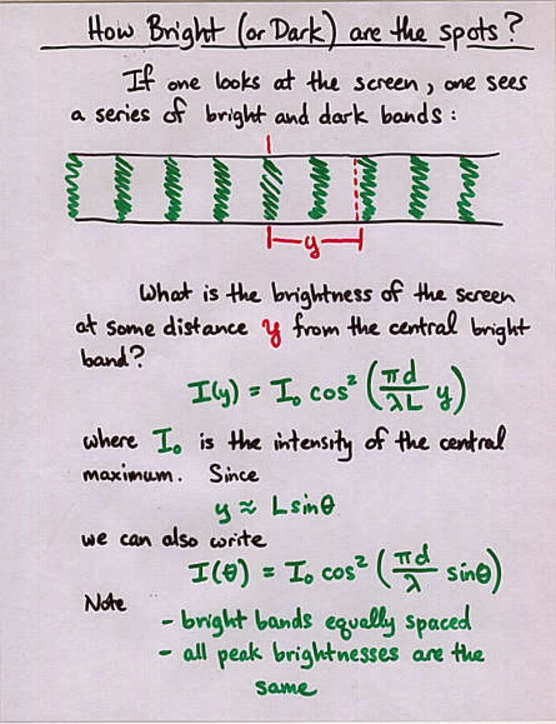Viewgraph 8A